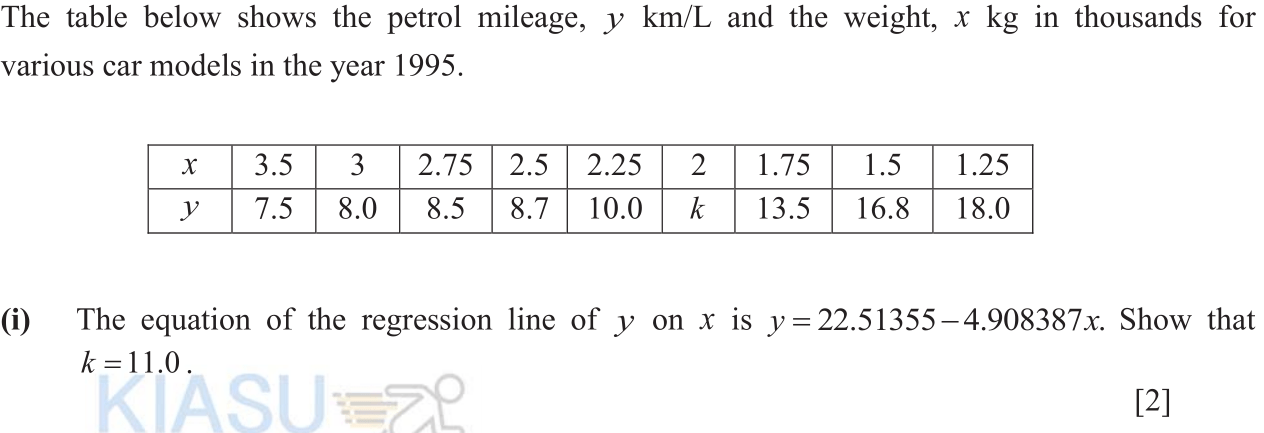# 25th July: Linear regression

## Recovering data points: using $(\overline{x}, \overline{y})$

By now, we should be familiar with substituting numbers into our regression lines to estimate values.

However, to recover an unknown data point, we cannot substitute values into our regression line because our regression line generally do not pass through the data points. This is where the theory that $(\overline{x}, \overline{y})$ lies on our regression lines come in.

### ExampleGiven $y=22.51355 – 4.908387x$, it is very tempting to sub in $x=2$ into the equation. We cannot do that. Instead, let’s calculate the mean $\overline{x}$. Now we can substitute this into the regression line to get $\overline{y}$. Finally we can work backwards to get $k$: give it a try.